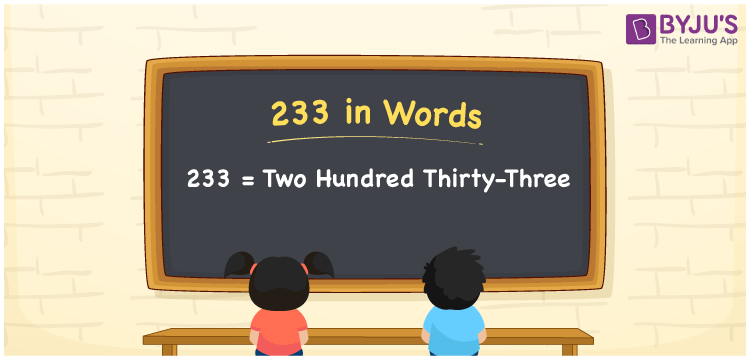# 233 in Words

233 in words is “two hundred thirty-three”. The number 233 has 3 digits, such as 3 in one’s place, 3 in ten’s place and 2 in hundred’s place. Thus, using the place values of 233, we can easily write the number 233 in words. In this article, we will discuss the method of writing the number 233 in words using the place value system in detail.

 233 in Words: Two Hundred Thirty-three. Two Hundred Thirty-three in Numerical Form: 233.

## 233 in English Words## How to Write 233 in Words?

Below is the place value table for 233, which is used to write the number 233 in words.

 Hundreds Tens Ones 2 3 3

The expanded form of 233 is as follows:

= 2 × Hundred + 3 × Ten + 3 × One

= 2 × 100 + 3 × 10 + 3 × 1

= 200 + 30 + 3

= 233

= Two hundred thirty-three

Hence, 233 in words is two hundred thirty-three.

233 in words – Two hundred thirty-three

Is 233 an odd number? – Yes

Is 233 an even number? – No

Is 233 a perfect square number? – No

Is 233 a perfect cube number? – No

Is 233 a prime number? – Yes

Is 233 a composite number? – No

## Frequently Asked Questions on 233 in Words

Q1

### How to spell 233 in words.

233 in words is two hundred thirty-three.

Q2

### Simplify 200 + 33, and express it in words.

Simplifying 200 + 33 we get 233. Hence, 233 in words is two hundred thirty-three.

Q3

### Is 233 a prime number?

Yes, 233 is a prime number.